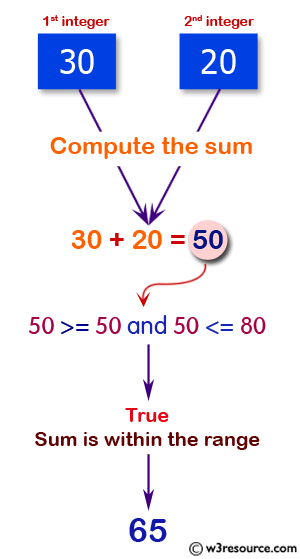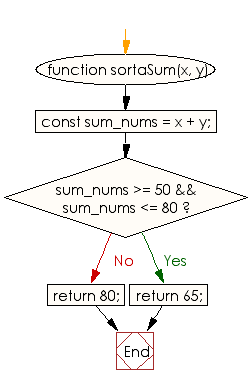# JavaScript: Compute the sum of the two given integers

## JavaScript Basic: Exercise-39 with Solution

Write a JavaScript program to compute the sum of the two given integers, If the sum is in the range 50..80 return 65 other wise return 80.

Pictorial Presentation:Sample Solution:

HTML Code:

``````<!DOCTYPE html>
<html>
<meta charset="utf-8">
<meta name="viewport" content="width=device-width">
<title>JavaScript program to compute the sum of the two given integers, If the sum is in the range 50..80  return 65 other wise return 80.</title>
<body>

</body>
</html>
```
```

JavaScript Code:

``````function sortaSum(x, y)
{
const sum_nums = x + y;
if (sum_nums >= 50 && sum_nums <= 80) {
return 65;
}
return 80;
}

console.log(sortaSum(30,20));
console.log(sortaSum(90,80));
```
```

Sample Output:

```65
80
```

Flowchart:ES6 Version:

``````function sortaSum(x, y)
{
const sum_nums = x + y;
if (sum_nums >= 50 && sum_nums <= 80) {
return 65;
}
return 80;
}

console.log(sortaSum(30,20));
console.log(sortaSum(90,80));
``````

Live Demo:

See the Pen JavaScript: compute the sum of the two given integers - basic-ex-39 by w3resource (@w3resource) on CodePen.

Improve this sample solution and post your code through Disqus

What is the difficulty level of this exercise?

Test your Programming skills with w3resource's quiz.

﻿

## JavaScript: Tips of the Day

Returns the sum of the powers of all the numbers from start to end (both inclusive)

Example:

```const sumPower = (end, power = 2, start = 1) =>
Array(end + 1 - start)
.fill(0)
.map((x, i) => (i + start) ** power)
.reduce((a, b) => a + b, 0);
console.log(sumPower(10)); // 385
console.log(sumPower(10, 3)); // 3025
console.log(sumPower(10, 3, 5)); // 2925
```

Output:

```385
3025
2925
```